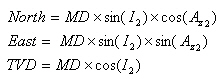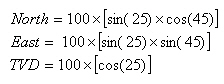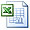# Tangential Method Calculation

The tangential method is the simplest calculation among other directional survey calculations.The tangential method formulas are listed below;Tangential Method Calculation formula

Where;

MD = Measured Depth between surveys in ft

I1 = Inclination (angle) of upper survey in degrees

I2 = Inclination (angle) of lower in degrees

Az1= Azimuth direction of upper survey

Az2 = Azimuth direction of lower survey

The following example is the Tangential Method Calculation.

Survey 1

Depth = 3500 ft

Inclination = 15 degree (I1)

Azimuth = 20 degree (Az1)

Survey 2

Depth = 3600 ft

Inclination = 25 degree (I2)

Azimuth = 45 degree (Az2)

Solution:

MD = 3600 – 3500 = 100 ftAs per the formulas above, you will get answers as listed below;

North = 29.88 ft

East = 29.88 ft

TVD = 90.63 ft

Please find the Excel sheet used for TheTangential Method Calculation

Share the joyWorking in the oil field and loving to share knowledge.

### 4 Responses to Tangential Method Calculation

1.chinonso says:

i acknowlegde this site for their work well done.

2.Steve Hopkins says:

There are several methods that can be used to calculate the survey data, of these some are accurate while are other may produce some error for a given situation.
Some of the most common methods used for survey calculation in the industry are:
Tangential method (Least Accurate)
Balanced Tangential method
Average Angle method
Radius of Curvature method (Most Accurate)
Minimum Curvature method (Most Accurate)

You’ve done a fine job, I thank you for sharing your knowledge.

3.manmohan says:

•DrillingFormulas.Com says: